Next: The PRP with retrieval Up: The Probability Ranking Principle Previous: The Probability Ranking Principle   Contents   Index

## The 1/0 loss case

We assume a ranked retrieval setup as in Section 6.3 , where there is a collection of documents, the user issues a query, and an ordered list of documents is returned. We also assume a binary notion of relevance as in Chapter 8 . For a query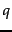and a document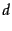in the collection, let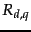be an indicator random variable that says whetheris relevant with respect to a given query. That is, it takes on a value of 1 when the document is relevant and 0 otherwise. In context we will often write just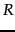for.

Using a probabilistic model, the obvious order in which to present documents to the user is to rank documents by their estimated probability of relevance with respect to the information need: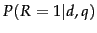. This is the basis of the Probability Ranking Principle (PRP) (van Rijsbergen, 1979, 113-114):

If a reference retrieval system's response to each request is a ranking of the documents in the collection in order of decreasing probability of relevance to the user who submitted the request, where the probabilities are estimated as accurately as possible on the basis of whatever data have been made available to the system for this purpose, the overall effectiveness of the system to its user will be the best that is obtainable on the basis of those data.''
In the simplest case of the PRP, there are no retrieval costs or other utility concerns that would differentially weight actions or errors. You lose a point for either returning a nonrelevant document or failing to return a relevant document (such a binary situation where you are evaluated on your accuracy is called 1/0 loss ). The goal is to return the best possible results as the top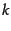documents, for any value ofthe user chooses to examine. The PRP then says to simply rank all documents in decreasing order of. If a set of retrieval results is to be returned, rather than an ordering, the Bayes Optimal Decision Rule , the decision which minimizes the risk of loss, is to simply return documents that are more likely relevant than nonrelevant: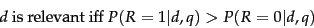(61)

Theorem. The PRP is optimal, in the sense that it minimizes the expected loss (also known as the Bayes risk ) under 1/0 loss.
End theorem.

The proof can be found in Ripley (1996). However, it requires that all probabilities are known correctly. This is never the case in practice. Nevertheless, the PRP still provides a very useful foundation for developing models of IR.Next: The PRP with retrieval Up: The Probability Ranking Principle Previous: The Probability Ranking Principle   Contents   Index